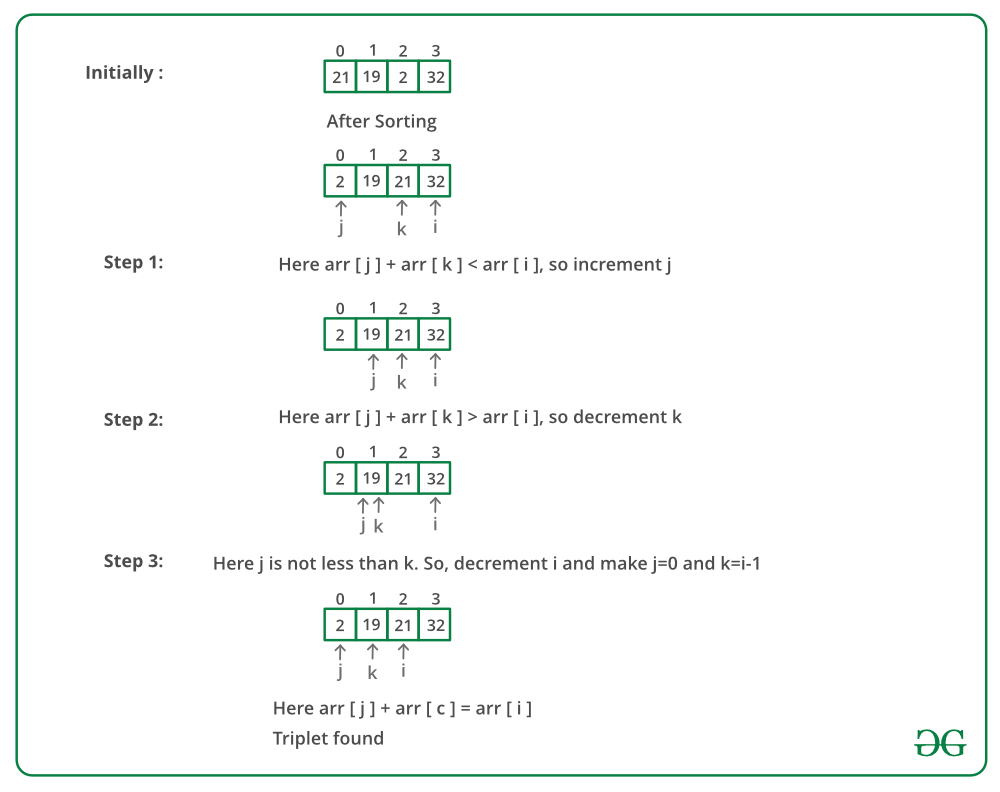Open In App

# Python Program to Find a triplet such that sum of two equals to third element

Given an array of integers, you have to find three numbers such that the sum of two elements equals the third element.
Examples:

```Input: {5, 32, 1, 7, 10, 50, 19, 21, 2}
Output: 21, 2, 19

Input: {5, 32, 1, 7, 10, 50, 19, 21, 0}
Output: no such triplet exist```

Question source: Arcesium Interview Experience | Set 7 (On campus for Internship)

Simple approach: Run three loops and check if there exists a triplet such that sum of two elements equals the third element.
Time complexity: O(n^3)
Efficient approach: The idea is similar to Find a triplet that sum to a given value.

• Sort the given array first.
• Start fixing the greatest element of three from the back and traverse the array to find the other two numbers which sum up to the third element.
• Take two pointers j(from front) and k(initially i-1) to find the smallest of the two number and from i-1 to find the largest of the two remaining numbers
• If the addition of both the numbers is still less than A[i], then we need to increase the value of the summation of two numbers, thereby increasing the j pointer, so as to increase the value of A[j] + A[k].
• If the addition of both the numbers is more than A[i], then we need to decrease the value of the summation of two numbers, thereby decrease the k pointer so as to decrease the overall value of A[j] + A[k].

Below image is a dry run of the above approach:Below is the implementation of the above approach:

## Python

 `# Python program to find three numbers``# such that sum of two makes the``# third element in array` `# Utility function for finding``# triplet in array``def` `findTriplet(arr, n):``    ` `    ``# Sort the array``    ``arr.sort()`` ` `    ``# For every element in arr check``    ``# if a pair exist(in array) whose``    ``# sum is equal to arr element``    ``i ``=` `n ``-` `1``    ``while``(i >``=` `0``):``        ``j ``=` `0``        ``k ``=` `i ``-` `1``        ``while` `(j < k):``            ``if` `(arr[i] ``=``=` `arr[j] ``+` `arr[k]):``               ` `                ``# Pair found``                ``print` `"numbers are "``, arr[i],``                       ``arr[j], arr[k]``                ``return``            ``elif` `(arr[i] > arr[j] ``+` `arr[k]):``                ``j ``+``=` `1``            ``else``:``                ``k ``-``=` `1``        ``i ``-``=` `1``        ` `    ``# No such triplet is found in array``    ``print` `"No such triplet exists"`` ` `# Driver code``arr ``=` `[``5``, ``32``, ``1``, ``7``, ``10``, ``50``, ``19``, ``21``, ``2``]``n ``=` `len``(arr)``findTriplet(arr, n)``# This code is contributed by Sachin Bisht`

Output:

`numbers are 21 2 19`

Time complexity: O(N^2)

Space Complexity: O(1) as no extra space has been used.

Please refer complete article on Find a triplet such that sum of two equals to third element for more details!